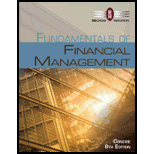# CAPM AND PORTFOLIO RETURN You have been managing a $5 million portfolio that has a beta of 1.25 and a required rate of return of 12%. The current risk-free rate is 5.25%. Assume that you receive another$500,000. If you invest the money in a stock with a beta of 0.75, what will be the required return on your $5.5 million portfolio?BuyFindarrow_forward ### Fundamentals of Financial Manageme... 8th Edition Eugene F. Brigham + 1 other Publisher: Cengage Learning ISBN: 9781285065137 #### Solutions Chapter SectionBuyFindarrow_forward ### Fundamentals of Financial Manageme... 8th Edition Eugene F. Brigham + 1 other Publisher: Cengage Learning ISBN: 9781285065137 Chapter 8, Problem 16P Textbook Problem 443 views ## CAPM AND PORTFOLIO RETURN You have been managing a$5 million portfolio that has a beta of 1.25 and a required rate of return of 12%. The current risk-free rate is 5.25%. Assume that you receive another $500,000. If you invest the money in a stock with a beta of 0.75, what will be the required return on your$5.5 million portfolio?

Summary Introduction

To determine: The required return.

The required rate of return:

The required rate of return is the minimum rate, which should be earned on an investment to keep that investment running in the market. When the required return is earned, only then the users and the companies invest in that particular investment.

### Explanation of Solution

Given,

The value of the portfolio is $5 million. The beta of the portfolio is 1.25. The required rate of return is 12%. The risk-free rate is 5.25%. The increased value of the portfolio is$5.5 million.

The beta of the portfolio is 0.75.

Calculated,

The new portfolio’s beta is 1.2 (working note).

The market risk premium is 9% (working note).

Calculation of the required rate of return:

The formula to calculate the required rate of return is,

ri=rRF+(rMrRF)×bi

Where,

• ri is the required return on the stock,
• rRF is the risk-free return,
• rM is the market risk premium and
• bi is the value of the stock’s beta.

Substitute 5.25% for rRF, 9% for rMrRF, and 1.2 for bi in the above formula.

ri=5.25%+9%×1.2=5.25%+10.8%=16.05%

The required return on the new portfolio is 16.05%.

Working note:

Calculation of the market risk premium:

(rMrRF)=rirRFbi=12%5

### Still sussing out bartleby?

Check out a sample textbook solution.

See a sample solution

#### The Solution to Your Study Problems

Bartleby provides explanations to thousands of textbook problems written by our experts, many with advanced degrees!

Get Started